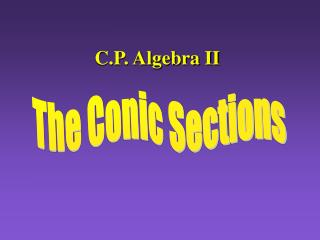DownloadDownload PresentationC.P. Algebra II

# C.P. Algebra II

Download Presentation## C.P. Algebra II

- - - - - - - - - - - - - - - - - - - - - - - - - - - E N D - - - - - - - - - - - - - - - - - - - - - - - - - - -
##### Presentation Transcript

1. C.P. Algebra II The Conic Sections

2. The Conic Sections Index The Conics Translations Completing the Square Classifying Conics

3. The Conics Parabola Ellipse Click on a Photo Hyperbola Circle Back to Index

4. The Parabola A parabola is formed when a plane intersects a cone and the base of that cone

5. Parabolas • A Parabola is a set of points equidistant from a fixed point and a fixed line. • The fixed point is called the focus. • The fixed line is called the directrix.

6. Parabolas Around Us

7. Parabolas Parabola FOCUS Directrix

8. To Find p Example: x2=24y 4p=24 p=6 4p is equal to the term in front of x or y. Then solve for p.

9. Examples for ParabolasFind the Focus and Directrix Example 1 y = 4x2 x2= (1/4)y 4p = 1/4 p = 1/16 FOCUS (0, 1/16) Directrix Y = - 1/16

10. Examples for ParabolasFind the Focus and Directrix Example 2 x = -3y2 y2= (-1/3)x 4p = -1/3 p = -1/12 FOCUS (-1/12, 0) Directrix x = 1/12

11. Examples for ParabolasFind the Focus and Directrix Example 3 (try this one on your own) y = -6x2 FOCUS ???? Directrix ????

12. Examples for ParabolasFind the Focus and Directrix FOCUS (0, -1/24) Example 3 y = -6x2 Directrix y = 1/24

13. Examples for ParabolasFind the Focus and Directrix Example 4 (try this one on your own) x = 8y2 FOCUS ???? Directrix ????

14. Examples for ParabolasFind the Focus and Directrix FOCUS (2, 0) Example 4 x = 8y2 Directrix x = -2

15. Parabola Examples Now write an equation in standard form for each of the following four parabolas

16. Write in Standard Form Example 1 Focus at (-4,0) Identify equation y2 =4px p = -4 y2 = 4(-4)x y2 = -16x

17. Write in Standard Form Example 2 With directrix y = 6 Identify equation x2 =4py p = -6 x2 = 4(-6)y x2 = -24y

18. Write in Standard Form Example 3 (Now try this one on your own) With directrix x = -1 y2 = 4x

19. Write in Standard Form Example 4 (On your own) Focus at (0,3) x2 = 12y Back to Conics

20. Circles A Circle is formed when a plane intersects a cone parallel to the base of the cone.

21. Circles

22. Standard Equation of a Circle with Center (0,0)

23. Circles & Points of Intersection Distance formula used to find the radius

24. CirclesExample 1 Write the equation of the circle with the point (4,5) on the circle and the origin as it’s center.

25. Example 1 Point (4,5) on the circle and the origin as it’s center.

26. Example 2Find the intersection points on the graph of the following two equations

27. Now what??!!??!!??

28. Example 2Find the intersection points on the graph of the following two equations Plug these in for x.

29. Example 2Find the intersection points on the graph of the following two equations Back to Conics

30. Ellipses

31. Ellipses Examples of Ellipses

32. Ellipses Horizontal Major Axis

33. FOCI (-c,0) & (c,0) CO-VERTICES (0,b)& (0,-b) The Equation Vertices (-a,0) & (a,0) CENTER (0,0)

34. Ellipses Vertical Major Axis

35. FOCI (0,-c) & (0,c) CO-VERTICES (b, 0)& (-b,0) The Equation Vertices (0,-a) & (0, a) CENTER (0,0)

36. Ellipse Notes • Length of major axis = a (vertex & larger #) • Length of minor axis = b (co-vertex & smaller#) • To Find the foci (c) use: c2 = a2 - b2

37. Ellipse ExamplesFind the Foci and Vertices

38. Ellipse ExamplesFind the Foci and Vertices

39. Write an equation of an ellipse whose vertices are (-5,0) & (5,0) and whose co-vertices are (0,-3) & (0,3). Then find the foci.

40. Back to the Conics

41. The Hyperbola

42. Hyperbola Examples

43. Asymptotes Vertices (a,0) & (-a,0) Foci (c,0) & (-c, 0) Hyperbola NotesHorizontal Transverse Axis Center (0,0)

44. Hyperbola NotesHorizontal Transverse Axis To find asymptotes

45. Vertices (a,0) & (-a,0) Asymptotes Foci (c,0) & (-c, 0) Hyperbola NotesVertical Transverse Axis Center (0,0)

46. Hyperbola NotesVertical Transverse Axis To find asymptotes